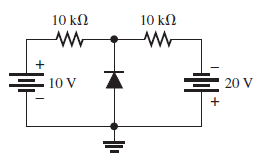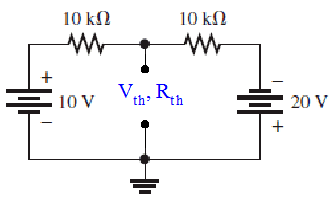# Diode two dc sources

## Homework Statement

:Find the current that go across the diode ans=0.86mA[/B]## Homework Equations

V=IR

3. The Attempt at a Solution
The Vt(v total) is 30v
so,must i ignore the diode as off first?.In that case the current is 30/20=1.5 mA.
i also know the that diode is foward bias because 20v>10V
i know that the voltage drop for this diode is 0.7
(20-0.7)/10=1.93,this step is correct.
but i really need help for this qn
mods pls dont delete.. :)

Last edited by a moderator:

NascentOxygen
Staff Emeritus
(20-0.7)/10=1.93,this step is correct.
That is the current for the 20V source. Also determine the current for the 10V source.

That is the current for the 20V source. Also determine the current for the 10V source.

hey but how can i just split the circuit into two?? lol, isist that weird?? like i used to learn that i should find the current and so on...

NascentOxygen
Staff Emeritus
You have made the assumption that the diode is conducting....which means you are saying its cathode must be at a potential of -0.7V.

So with this assumption, you can then determine the current in each resistor.

http://imgur.com/l40uOZ2
http://imgur.com/l40uOZ2
the red arrow shows the current flow from the 10v source.No current from the 10V pass am I right??

NascentOxygen
Staff Emeritus
What does Ohm's Law tell you for the resistor on the left, given your assumption that the diode's cathode is being held at -0.7V?

hey thats is the part i dont understand.. i really dont :(

NascentOxygen
Staff Emeritus
hey thats is the part i dont understand.. i really dont :(
You have a resistor, you know how many ohms, and you also know the voltage at either end of it ....

but shouldn't it be like (30-0.7 )/20????

gneill
Mentor
nathanxx, why don't you make things simpler for yourself? ... If you are studying diode circuits you very likely have already covered Thevenin equivalents. If you consider the diode as the load of the network, what would be the Thevenin equivalent of the circuit that is driving that load?NascentOxygen
Staff EmeritusFrom their difference you can deduce how much current must be going through the diode.